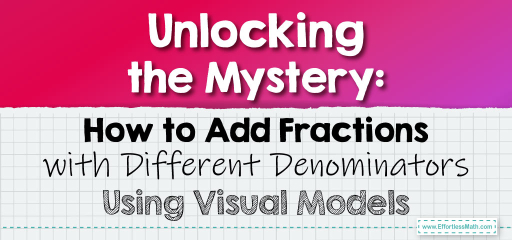# Unlocking the Mystery: How to Add Fractions with Different Denominators Using Visual Models

Adding fractions with unlike denominators might seem like a daunting task at first, but with the right approach and understanding, it becomes a breeze.One of the most effective ways to grasp this concept is by using visual models. In this blog post, we’ll explore a step-by-step guide on how to add fractions with different denominators using models, ensuring you have a solid foundation in this essential grade 5 math topic.

## Step-by-step Guide:

1. Understanding the Basics:

Before diving into adding fractions with unlike denominators, it’s crucial to understand what a fraction represents. A fraction consists of a numerator (the top number) and a denominator (the bottom number). The denominator tells us into how many equal parts a whole is divided, while the numerator indicates how many of those parts we have.

2. Identifying Unlike Denominators:

If two fractions have different denominators, they have unlike denominators. For example, in the fractions $$\frac{1}{3}$$ and $$\frac{2}{5}$$, the denominators 3 and 5 are different.

3. Using Models for Visualization:

Draw two separate models (like circles or rectangles) and divide them based on the denominators of the fractions you’re adding. For our example, one model will be divided into 3 equal parts and the other into 5 equal parts.

4. Finding a Common Denominator:

To add fractions with unlike denominators, we need a common denominator. This is a number that both denominators can divide into. In our example, the least common denominator for 3 and 5 is 15.

Using the models, adjust the fractions to have a common denominator. For our example, $$\frac{1}{3}$$ becomes $$\frac{5}{15}$$ and $$\frac{2}{5}$$ becomes $$\frac{6}{15}$$.

Now, simply add the numerators of the adjusted fractions. Using our example, $$5 + 6 = 11$$. So, $$\frac{1}{3} + \frac{2}{5} = \frac{11}{15}$$.

### Examples 1:

Add $$\frac{2}{4}$$ and $$\frac{3}{8}$$ using models.

Solution:

The least common denominator is 8. Adjusting the fractions, $$\frac{2}{4}$$ becomes $$\frac{4}{8}$$. So, $$\frac{2}{4} + \frac{3}{8} = \frac{7}{8}$$.

The Absolute Best Book for 5th Grade Students

### Example 2:

Add $$\frac{3}{6}$$ and $$\frac{1}{3}$$ using models.

Solution:

The least common denominator is 6. The fraction $$\frac{3}{6}$$ remains the same, while $$\frac{1}{3}$$ becomes $$\frac{2}{6}$$. So, $$\frac{3}{6} + \frac{1}{3} = \frac{5}{6}$$.

### Practice Questions:

1. Add $$\frac{1}{5}$$ and $$\frac{2}{10}$$ using models.

2. Add $$\frac{3}{7}$$ and $$\frac{2}{14}$$ using models.

3. Add $$\frac{4}{9}$$ and $$\frac{2}{3}$$ using models.

A Perfect Book for Grade 5 Math Word Problems!

1. $$\frac{3}{10}$$

2. $$\frac{4}{7}$$

3. $$\frac{10}{9}$$ or $$1 \frac{1}{9}$$

The Best Math Books for Elementary Students

### What people say about "Unlocking the Mystery: How to Add Fractions with Different Denominators Using Visual Models - Effortless Math: We Help Students Learn to LOVE Mathematics"?

No one replied yet.

X
51% OFF

Limited time only!

Save Over 51%

SAVE $15 It was$29.99 now it is \$14.99